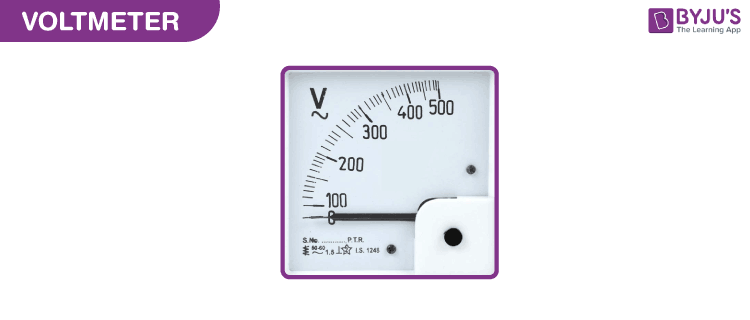# Types of Voltmeter

The types of voltmeters are based on the construction principle and types of measurements.## Based on the construction principle:

• Permanent Magnet Moving Coil Voltmeter: It is also known as the D’Arsonval meter and is used for measuring the current flowing through the coil by determining the angular deflection in the uniform magnetic field.
• Moving Iron Voltmeter: There are two types of moving iron voltmeter, and they are attraction type and repulsion type.
• Electro Dynamometer Type Voltmeter: When a current-carrying conductor is placed inside a magnetic field, the conductor experiences a mechanical force which is the cause of deflection in the conductor.
• Rectifier Type Voltmeter: This is used for measuring the alternating voltage and current.
• Induction Type Voltmeter: These voltmeters are the most commonly used voltmeter as their construction is easy.
• Electrostatic Type Voltmeter: The deflecting torque takes place with the help of a static electrical field. These voltmeters are used for the measurement of high voltage.
• Digital Voltmeter: Digital voltmeter is used in place of analogue voltmeter because digital voltmeter displays AC and DC voltage value and is used as discrete numerical instead of pointer deflection.

• DC Voltmeter
• AC Voltmeter

## Difference between DC and AC voltmeter

• The DC voltmeter measures the DC voltage, but the AC voltmeter measures the AC voltage.
• The DC voltmeter measures the peak value of DC voltage, but the AC voltmeter measures the RMS value of the AC voltage.
• DC voltmeter does not use a rectifier before a multistage amplifier, whereas the  AC voltmeter measures the RMS value of the AC voltage.

### Refer more(9)(1)